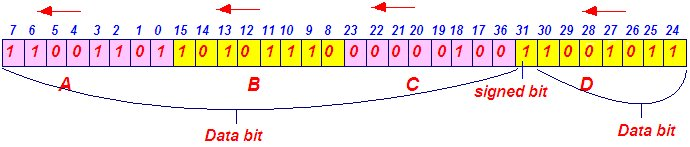## INDEX

### Memory representation of long int

Memory representation of unsigned long int: unsigned long inta=8888855555;

It is 32-bit data type and all its 32 bit is data bit.

Binary equivalent of 888885555 is: 110100 11111011 01010001 00110011
For 32 bit we will add 2 zero in the left side i.e. 00110100 11111011 01010001 00110011. Here

A is 00110100
B is 11111011
C is 01010001
D is 00110011

Memory representation:2. Memory representation of signed long int:

For positive number: long int a=8888855555 or signed long int a=8888855555;

It is 32 bit data type.
It of 8888855555 is 110100 11111011 01010001 0011001131
Bit: data bit
Binary equivalent
1 bit: signed bit

For 16 bit we will add 2 zero in the left side i.e 00110100 11111011 01010001 00110011. Here
A is 00110011
B is 01010001
C is 11111011
D is 00110100

Memory representation:

For negative number: long int a= -8888855555 or signed long int a= -8888855555;

It is 32 bit data type.
Bit no 31 is signed bit.
855555 is 110100 11111011 01010001 00110011

For 32 bit we will add 2
` `
`Binary equivalent of 8888 zero in the left side i.e. 00110100 11111011 01010001 00110011`

Negative numbers are converted in 2's complement format before stored in the memory.
Since a is negative number so it will first convert in the 2’so

1’s Complement of a:11001011 00000100 10101110 11001100
+                                1
___________________________________
2’s Complement of a: 11001011 00000100 10101110 11001101

Memory representation: